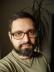## Perl Weekly Challenge 005: The Anagrams

When I read the definition of an anagram in Wikipedia, I knew I would need a combinatorics module. I first checked Math::Combinatorics and tried to generate all the anagrams using its `next_permutation` method. I could have used Algorithm::Combinatorics and its `permutations`, as well.

But there was a catch: if a letter is repeated in the input word, the anagrams won’t be unique. I didn’t want to reach for List::Util’s `uniq` as it needs to keep all the anagrams in memory while the iterator had much lower memory footprint. Fortunately, I knew that “unique” means “hash” in Perl.

``````#!/usr/bin/perl
use warnings;
use strict;
use feature qw{ say };

use Math::Combinatorics;

my @letters = split //, shift;
my \$iter = 'Math::Combinatorics'->new(data  => \@letters);
my %seen;
\$seen{\$_}++ or say \$_ while \$_ = join '', \$iter->next_permutation;
``````

## Real Words

The common meaning of “anagram” is different, though: it only means real meaningful words that are formed using the letters the original word consists of. For example, fluster is an anagram of restful, but not rlftsue. I happen to have a list of many (more than 300K) English words in the file `/usr/share/dict/british` on my system, so I used it to filter the anagrams:

``````#!/usr/bin/perl
use warnings;
use strict;
use feature qw{ say };

use Math::Combinatorics;

my @letters = split //, shift;

my %seen;
open my \$dictionary, '<', '/usr/share/dict/british' or die \$!;
chomp, --\$seen{\$_} while <\$dictionary>;

my \$iter = 'Math::Combinatorics'->new(data  => \@letters);
++\$seen{\$_} or say \$_ while \$_ = join '', \$iter->next_permutation;
``````

I intentionally changed the original program the least I could. Can you explain why the `++` was moved?

## Most Anagrams

To solve the second challenge, we don't need to generate all the possible anagrams and count them. Playing with simple samples I was able to find the formula:

n = length ! / Πc(freq(c) !)

or, in other words, the number of anagrams equals the factorial of the length of the word divided by the product of factorials of all the frequencies of its letters.

``````#!/usr/bin/perl
use warnings;
use strict;
use feature qw{ say };

sub factorial {
my (\$n) = @_;
\$n *= \$_ for 2 .. \$n - 1;
return \$n
}

my @max = (0, "");
while (my \$word = <>) {
chomp \$word;
my %frequency;
++\$frequency{\$_} for my @letters = split //, \$word;
my \$count = factorial(scalar @letters);
\$count /= factorial(\$frequency{\$_}) for keys %frequency;
@max = (\$count, \$word) if \$count > \$max;
}

say "@max";
``````

## Long Words

For longer words, the factorial gets very large and can become a float, causing imprecise results. For `"a" x 100 . "b" x 100` the program returns `inf`. We can reach for Math::BigInt to fix that. Just replace the first line in the factorial subroutine with
``my \$n = 'Math::BigInt'->new(shift);``I blog about Perl.